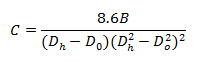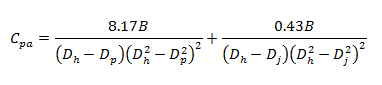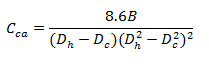# Coefficient For Annulus Around Drill Pipe and Drill Collar

A term “C” represents the general coefficient for annulus around drill pipe and drill collar and the equation is as follow:Where:

Dh = hole diameter, inch

Do = Outside diameter of drill pipe or drill collar, inch

B = a parameter which takes into account the difference between Q1.86 and Q2

This term does not consider the different between size of tool joint and pipe which affect pressure drop in the annulus. This topic will demonstrate equations to get more accurate coefficient.

Coefficient of Annulus around Drill Pipe and Tool Joint

In order to get an accurate annulus pressure drop around a drill string, the coefficient for the annulus around tool joint must be taken in to account.With the tool joint adjustment, the equation of coefficient of annulus around drill pipe and tool joint is shown in the following equation.Where:

Cpa = coefficient for the annulus of pipe

Dh = hole diameter, inch

Dp = outside diameter of drill pipe, inch

Dj = outside diameter of tool joint, inch

B = a parameter which takes into account the difference between Q1.86 and Q2. You can just simply select “B” value from the following table.Coefficient of Annulus around Drill Collar

Since OD of tool joint of drill collar is the same size as OD of drill collar therefore there is nothing change regarding the coefficient.The equation is show below:Where:

Cca = coefficient for the annulus of drill collar

Dh = hole diameter, inch

Dc = outside diameter of drill collar, inch

B = a parameter which takes into account the difference between Q1.86 and Q2

Reference:Drilling Hydraulic Books

Share the joy